• 交换数组中两个元素位置方法一:苹果提供的swap方法代码var originArrayOne = [2,3,4] print("originArrayOne排序前:\(originArrayOne)") //交换第0个元素和第2个元素位置 //方法一 swap(&originArrayOne, &...
交换数组中两个元素位置
方法一:苹果提供的swap方法
代码
var originArrayOne = [2,3,4]
print("originArrayOne排序前:\(originArrayOne)")
//交换第0个元素和第2个元素位置
//方法一
swap(&originArrayOne, &originArrayOne)
print("originArrayOne排序后:\(originArrayOne)\n")
效果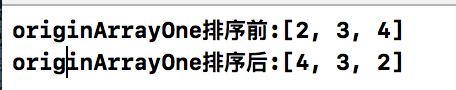方法二: 使用元祖
代码:
//方法二
var originArrayTwo = [2,3,4]
print("originArrayTwo排序前:\(originArrayTwo)")
(originArrayTwo,originArrayTwo) = (originArrayTwo,originArrayTwo)
print("originArrayTwo排序后:\(originArrayTwo)\n")

效果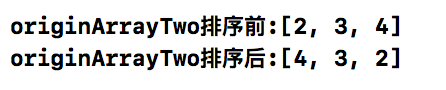方法三: 自定义swap(方法一的实现)
代码:
//方法三(方法一的实现)
var originArrayThree = [2,3,4]
print("originArrayThree排序前:\(originArrayThree)")
mySwap(&originArrayThree,&originArrayThree)
print("originArrayThree排序后:\(originArrayThree)")
func mySwap<T>(_ i : inout T, _ j : inout T){
var temp = i
i = j
j = temp
}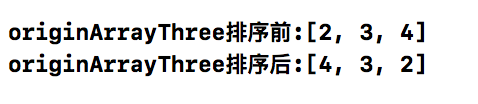还有更多更好的方法请联系我
展开全文swift 苹果
• 简单的交换数组中个元素位置，{ 8，12 }交换后为{ 12，8 }. 同样交换俩个数字，比如这样的代码 public static void swap(int a, int b) { int t = a; a = b; b = t; } 然后再打印的话，实际上是交换不了的。...
简单的交换数组中俩个元素的位置，{ 8，12 }交换后为{ 12，8 }. 同样交换俩个数字，比如这样的代码
public static void swap(int a, int b) {
int t = a;
a = b;
b = t;
}
然后再打印的话，实际上是交换不了的。因为此时的 a, b只是形参，我们知道形参的改变不会影响实参的变化，因此交换不了。那么为什么数组可以交换呢，让我们来看一下。
public static void swap(int[] arr,int i, int j) {
int t = arr[i];
arr[i] = arr[j];
arr[j] = t;
}

public static void main(String[] args) {
int[] array = { 8, 12 };
System.out.println(Arrays.toString(array));
swap(array, 0, 1);
System.out.println(Arrays.toString(array));
}
在打印时，首先定义了一个数组，因此在栈中申请了一个栈帧，此时的array是指向{ 8， 12 }的。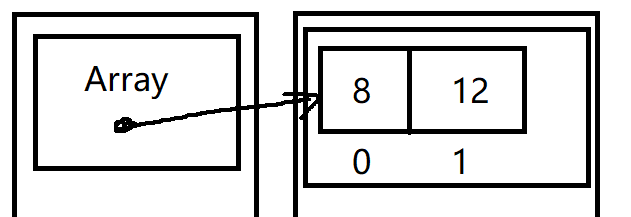第二次打印时，调用了swap方法，array此时还是指向{ 8，12 }，此时{ 8，12 }的下标为 0 和 1 ，然后arr[ i ] 的值赋给 t ，t 此时为8，arr[j] 的值赋给 arr[i]，arr[i]的值此时为12，最后 t 的值赋给arr[i]，此时arr[j]为8，这就完成了俩个值的交换。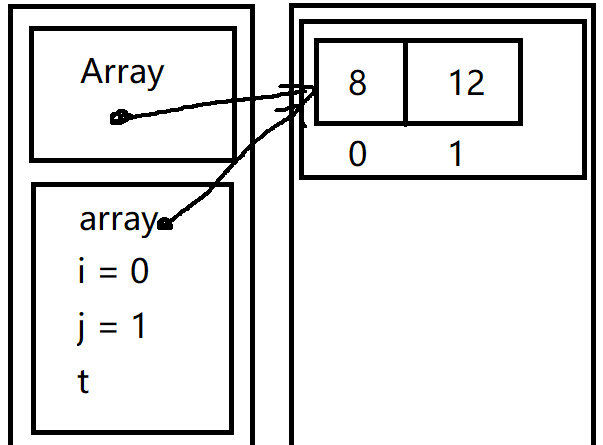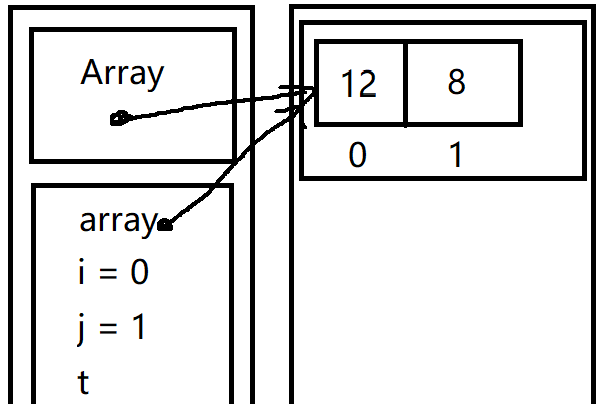最后完成交换之后，array这个栈帧就完成了他的任务，会被消亡移出栈，可以看到此时Array指向的数组变成了{ 12，8 }。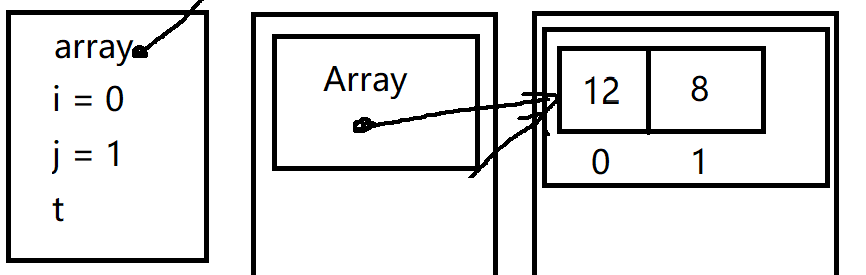最后是编译运行的一个结果：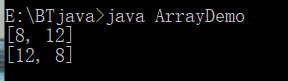这样，就简单的完成了数组中俩个元素值的交换。 同时，我们还需要记住形参的改变不会影响实参的改变这句话。
展开全文Java
• 方法一 public static void swap(int[] arr,int i,int j){ arr[i]=arr[i]^arr[j]; arr[j]=arr[i]^arr[j]; arr[i]=arr[i]^arr[j]; ... public static void swap(int[] arr,int i,int j){ ...
方法一


public static void swap(int[] arr,int i,int j){
arr[i]=arr[i]^arr[j];
arr[j]=arr[i]^arr[j];
arr[i]=arr[i]^arr[j];

}

方法二
    public static void swap(int[] arr,int i,int j){
int temp=arr[i];
arr[i]=arr[j];
arr[j]=temp;
}

展开全文数据结构 算法 java
• 在公司业务碰到的 一些需求 1.将数组的一个元素 置顶操作 function changeToFirstLocation...交换数组中两个元素位置 // 删除数组的元素 并插入新的数值 将删除的元素组成一个数组返回splice(index,count,new
在公司中业务中碰到的 一些需求
1.将数组中的一个元素 置顶操作
function changeToFirstLocation(arr,index){
return arr.unshift(arr.splice(index,1));
//  或者
var tmp=arr;
arr=arr[index];
arr[index]=tmp;
}

交换数组中两个元素的位置 // 删除数组中的元素 并插入新的数值 将删除的元素组成一个数组返回splice(index,count,newValue); index 开始删除元素的下标 count 从当前下标 往后面 删除几个 newValue 替代删除位置的新元素
// 两个元素交换位置
function changeLocation1(arr,index1,index2){
arr[index1-1]=arr.splice(index2-1,1,arr[index1-1]);

return arr;
}


3.位置移动 后面元素移动到某个位置 从那个位置开始后面的元素都往后面移动一位 index1 移动到 index2 将 index2的位置 占住
function changeLocation2(arr,index1,index2){
var left,middle,right;
if(index1 == index2) {
return arr;
}
middle=arr.splice(index1,1);
// 判断是向前还是向后移动
if(index1<index2){   // 向后移动
left=arr.filter((item,index)=>{
return index<=index2;
});

right=arr.filter((item,index)=>{
return index>index2;
})

} else {
left=arr.filter((item,index)=>{
return index<index2;
});

right=arr.filter((item,index)=>{
return index>=index2;
})
}
return [...left,middle,...right];
}

关注我 持续更新前端知识 关注冲哥
展开全文javascript
• 【解题核心】：在Java交换数组两个元素下标位置，必须通 过引用来交换。而不能直接进行交换 【代码演示】： public class Swap { public static void swap(int[] a, int i, int j) { int t = a[i]; a[i] ...
• 任意交换数组中两个元素，使得有序的最小交换次数 import java.util.*; public class Main{ public static void main(String[] args) { Scanner scanner = new Scanner(System.in); while (scanner.hasNextInt...
• static void swap(int[] array, int index1,int index2){ int t = array[index1]; array[index1] = array[index2]; array[index2] = t; }java 基础 算法
• 假设将一个数组的首尾互换一下，利用数组的 splice 替换元素方法很轻松就办到。 var arr = [ { id: 0, name: 'Rose' }, { id: 1, name: 'Robin' }, { id: 2, name: 'Tom' } ] var sourceIndex = 0 var...
• 该楼层疑似违规已被系统折叠隐藏此楼查看此楼#include #include #include int main(void){int a[]={1,2,3,4,5,6,7,8};int b[]={9,10,11,12,13,15};int lena,lenb,randa,randb,randtimes;int i,temp;srand((unsigned)...
• arr[oneIndex] = [arr[twoIndex], arr[twoIndex] = arr[oneIndex]] 更简单的：let x = 1let y = 2[x, y] = [y, x]
• 这次牛客那个CSL 的魔法那个题用到了这知识也是第一次知道 直接模板得了 int getMinSwaps(vector<int> &nums){ //排序 vector<int> nums1(nums); sort(nums1.begin(),nums1.end()); unordered...
• 原始数据：数组中三个关于电影的对象数据 const movies = [ { title: 'a', year: 2018, rating: 4. 3 }, { title: 'b', year: 2018, rating: 4.7 }, { title: 'c', year: 2018, rating: 4.9 } ]; 需求：需要...es6
• 例题：请补充fun函数，该函数的功能是：交换数组a最大和最小两个元素位置，结果重新保存在原数组，其它元素位置不变。注意数组a没有相同元素。 例如，输入 “20,40,78,60,52”,则输出结果为 “78，40，20，...
• 在拖拽等操作，我们经常会操作数组，包括交换两个数组位置。核心思想是利用splice的删除、新增、替换来实现，splice会改变原数组，所以最好在操作之前进行一下拷贝。splice会返回一个新的数组，删除的时候会返回...
• swap函数用来交换数组中两个值 public void swap(int[] data,int num1, int num2) { data[num1] = data[num1] ^ data[num2]; data[num2] = data[num1] ^ data[num2]; data[num1] = data[num1] ^ data[num2]; ...java 算法
• 给你一个数组两个索引，交换下标为这两个索引的数字 样例 样例 1: 输入: [1, 2, 3, 4], index1 = 2, index2 = 3 输出: 交换后你的数组应该是[1, 2, 4, 3]， 不需要返回任何值，只要就地对数组进行交换即可。 ...
• // 假设要交换第2个和第4个元素 let x = 2, y = 4; arr.splice(x - 1, 1, ...arr.splice(y - 1, 1, arr[x - 1])) console.log(arr) /// [1,2,4,3,5,6] // splice 向数组中添加, 删除元素, 返回值为被删除元素的...js
• ES6特性的一些应用 数组解构实现数组元素位置交换 若要交换i,j的位置只需要[a[i],a[j]] = [a[j],a[i]],一行搞定很酷。 var a = [1,2,3]; [a,a] = a[a,a]; console.log(a); //[3,2,1]; ...es6 array
• 端午假期写一道算法题的时候就栽在数组元素交换上了。开始的时候写了一用于交换的函数： public static void swap(int x,int y){ int z = x; x = y; y = z; }没什么技术含量，就是以前经常使用的借助一...Java 引用
• 定义一个数组，用函数交换数组中两个元素 #include <stdio.h> void swap(int *a, int *b); int main(void) { int Array[]={1,2,3,4,5}; swap(Array+1, Array+3); for(int i=0; i<5; i++){ printf("%...c语言 指针
• def swap2(a: Array[Int]) = a match { case Array(a, b, end@_*) =&...assert(swap2(Array(1, 2, 3, 4)).deep == Array(2, 1, 3, 4).deep) 一大大的彩蛋：https://mp.csdn.net/postedit/82686...
• 描述给你一个数组两个索引，交换下标为这两个索引的数字您在真实的面试是否遇到过这个题？ 是样例给出 [1,2,3,4] index1 = 2, index2 = 3. 交换之后变成 [1,2,4,3]public class Solution { /** * @param......